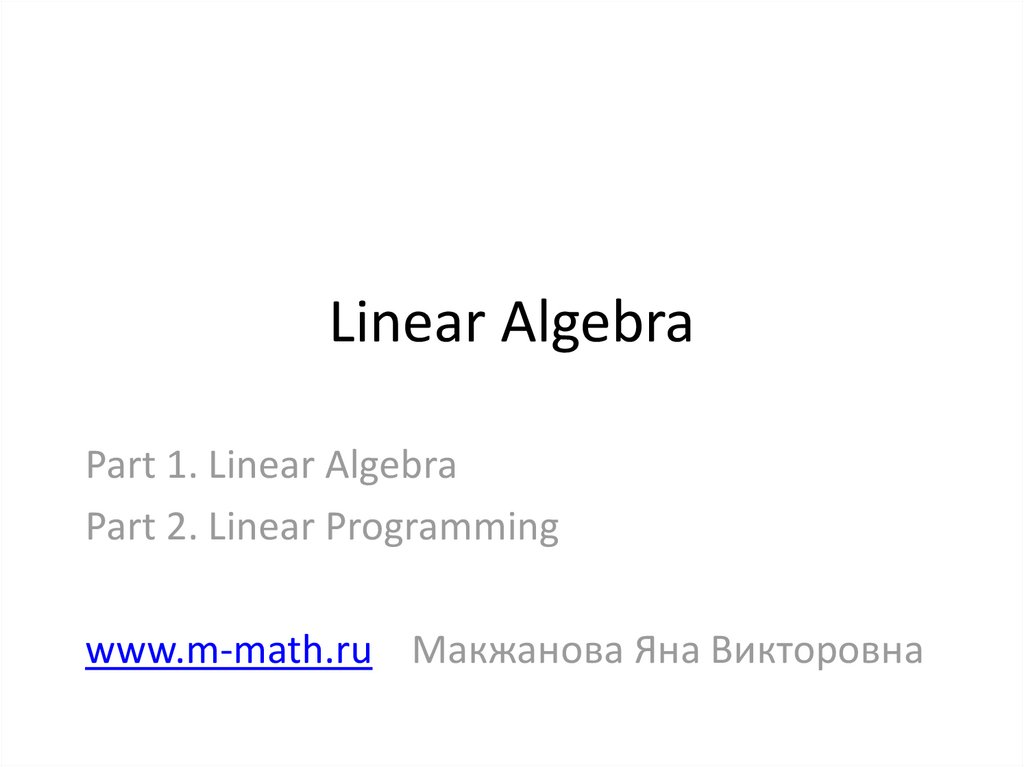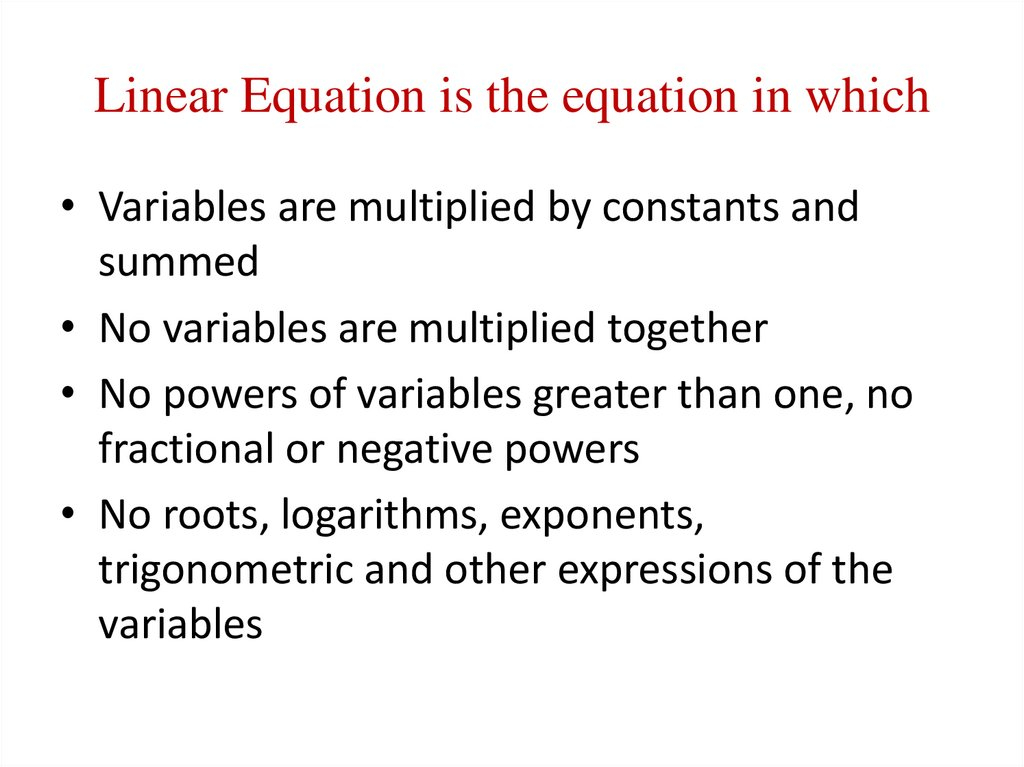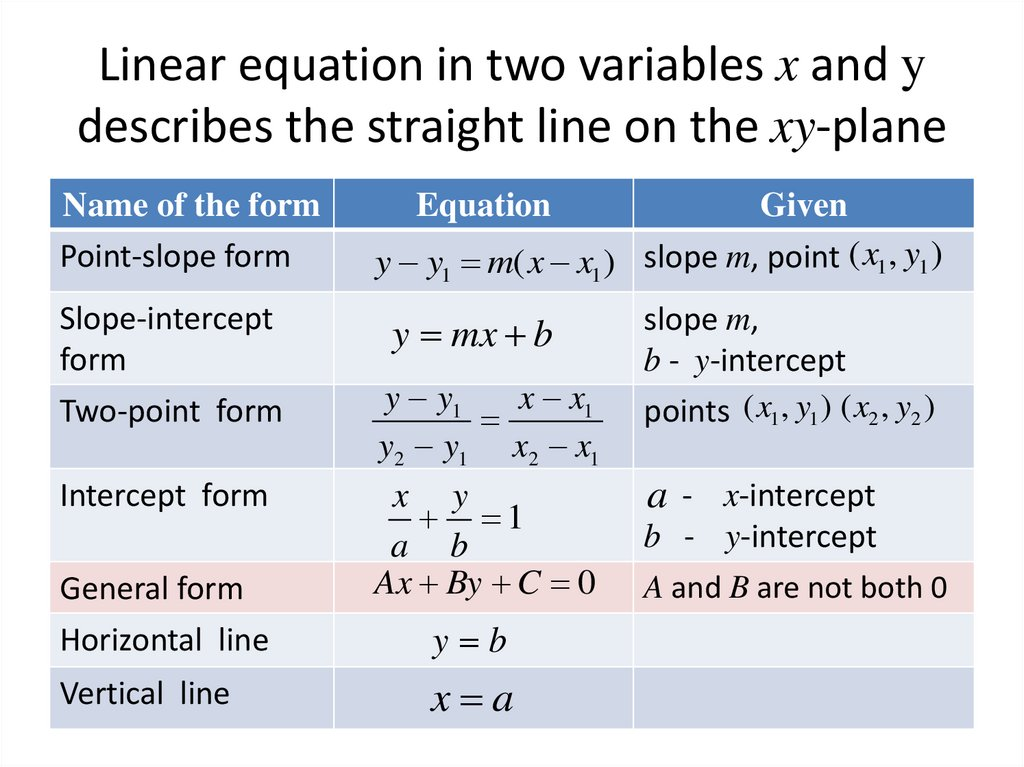# Straight lines and Linear Equations

## 1. Linear Algebra

Part 1. Linear Algebra
Part 2. Linear Programming
www.m-math.ru Макжанова Яна Викторовна

## 2. Linear Equation is the equation in which

• Variables are multiplied by constants and
summed
• No variables are multiplied together
• No powers of variables greater than one, no
fractional or negative powers
• No roots, logarithms, exponents,
trigonometric and other expressions of the
variables

## 3. Linear equation in two variables x and y describes the straight line on the xy-plane

Name of the form
Point-slope form
Slope-intercept
form
Two-point form
Intercept form
General form
Horizontal line
Vertical line
Equation
Given
y y1 m( x x1 ) slope m, point ( x1, y1 )
y mx b
y y1
x x1
y2 y1 x2 x1
x y
1
a b
Ax By C 0
y b
x a
slope m,
b - y-intercept
points ( x1, y1 ) ( x2 , y2 )
a - x-intercept
b - y-intercept
A and B are not both 0

## 4. Parallel and perpendicular

• Two straight lines with slopes m1 and m2 are
parallel if m1=m2.
• Two straight lines with slopes m1 and m2 are
perpendicular if m1m2= -1.

## 6. Linear Cost Model

Total Costs = Variable Costs + Fixed Costs
depend on the level
of production
TC = m·x + b
variable costs
per unit
amount of
commodity
produced
do not depend on the
level of production
Linear Cost Model
fixed costs

## 7. Break-even Analysis

• If TC > TR
• If TC < TR
• If TC = TR
a loss
a profit
no loss and no profit

## 8. Supply and Demand

• Law of Supply - a relation specifying the
amount of any commodity that manufacturers
(or sellers) can make available in the market at
various prices.
• Law of Demand - a relationship that
specifies the amounts of a particular
commodity that consumers are willing to buy
at various price levels.

## 9. Linear Supply and Demand Laws

• S: p mS x bS
• D: p mD x bD
x – amount of commodities
p – price for one commodity